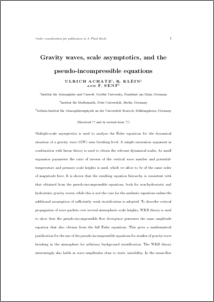Repository: Freie Universität Berlin, Math Department

# Gravity waves, scale asymptotics, and the pseudo-incompressible equations

Achatz, U. and Klein, R. and Senf, F. (2010) Gravity waves, scale asymptotics, and the pseudo-incompressible equations. Journal of Fluid Mechanics, 663 . pp. 120-147.Preview

260kB

## Abstract

Multiple-scale asymptotics is used to analyze the Euler equations for the dynamical situation of a gravity wave (GW) near breaking level. A simple saturation argument in combination with linear theory is used to obtain the relevant dynamical scales. As small expansion parameter the ratio of inverse of the vertical wave number and potentialtemperature and pressure scale heights is used, which we allow to be of the same order of magnitude here. It is shown that the resulting equation hierarchy is consistent with that obtained from the pseudo-incompressible equations, both for non-hydrostatic and hydrostatic gravity waves, while this is not the case for the anelastic equations unless the additional assumption of sufficiently weak stratication is adopted. To describe vertical propagation of wave packets over several atmospheric scale heights, WKB theory is used to show that the pseudo-incompressible flow divergence generates the same amplitude equation that also obtains from the full Euler equations. This gives a mathematical justication for the use of the pseudo-incompressible equations for studies of gravity-wave breaking in the atmosphere for arbitrary background stratication. The WKB theory interestingly also holds at wave amplitudes close to static instability. In the mean-flow equations we obtain in addition to the classic wave-induced momentum-flux divergences a wave-induced correction of hydrostatic balance in the vertical-momentum equation which cannot be obtained from Boussinesq or anelastic dynamics.

Item Type: Article Mathematical and Computer Sciences > Mathematics > Applied Mathematics Department of Mathematics and Computer Science > Institute of Mathematics > Geophysical Fluid Dynamics Group 888 Ulrike Eickers 25 Mar 2010 14:25 03 Mar 2017 14:40

Repository Staff Only: item control page